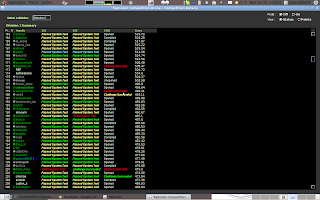## Thursday, December 29, 2011

### SRM528

Level 1 Level 2 Level 3
Div 1 Level 1 Level 2 Level 3
Div 2 Level 1 Level 2 Level 3SRM528

## Tutorials:

### Source Code:

/**
* Dynamic Programming.
*
* Let dp[i][j] represents the number of eels of length exactly 10 she can produce
* with the first i included elements and j cuts.
*
* dp[i][j] = Math.max(dp[i][j], dp[i - 1][j - k] + tmp);
* k is the cuts could be implemented on the i-th.
* dp[i-1][j-k] represents the maximum number could be produced with first i-1 th elements
* and j-k cuts. tmp is the number produced on ith element with k cuts.
*
*/

/**
* @author antonio081014
* @since Dec 28, 2011, 8:24:07 AM
*/
import java.util.Arrays;

public class Cut {
public int getMaximum(int[] eelLengths, int maxCuts) {
int N = eelLengths.length;
int ret = 0;
int[][] dp = new int[N + 1][maxCuts + 1];
Arrays.sort(eelLengths);
for (int i = 1; i <= N; i++) {
int cuts = (int) ((eelLengths[i - 1] - 1) / 10);
for (int j = 0; j <= maxCuts; j++) {
for (int k = 0; k <= Math.min(j, cuts); k++) {
int tmp = 0;
if (k == 0)
tmp = eelLengths[i - 1] == 10 ? 1 : 0;
else if (k == cuts)
tmp = (int) Math.floor(eelLengths[i - 1] / 10);
else
tmp = k;
dp[i][j] = Math.max(dp[i][j], dp[i - 1][j - k] + tmp);
ret = Math.max(ret, dp[i][j]);
}
}
}
return ret;
}
// <%:testing-code%>
}

### Source Code:

/**
* Straight implementation.
*
* Go through bi-directional on the same time.
* When the two characters are both '?', then add the smaller cost of oCost and xCost.
* When the one of two characters is '?', then add the corresponding cost.
* When none of them are '?', check if they are in the same character.
*/

/**
* @author antonio081014
* @since Dec 28, 2011, 8:17:29 AM
*/

public class MinCostPalindrome {
public int getMinimum(String s, int oCost, int xCost) {
int cost = 0;
for (int i = 0, j = s.length() - 1; i < j; i++, j--) {
if (s.charAt(i) == '?' && s.charAt(j) == '?')
cost += 2 * Math.min(oCost, xCost);
else if (s.charAt(i) == '?')
cost += (s.charAt(j) == 'x' ? xCost : oCost);
else if (s.charAt(j) == '?')
cost += (s.charAt(i) == 'x' ? xCost : oCost);
else if (s.charAt(i) != s.charAt(j))
return -1;
}
return cost;
}
// <%:testing-code%>
}